SSC BOARD PAPERS IMPORTANT TOPICS COVERED FOR BOARD EXAM 2024

### Practice Set 1.2 Similarity Class 10th Mathematics Part 2 MHB Solution

Practice Set 1.2

1. Given below are some triangles and lengths of line segments. Identify in which figures,…
2. In Δ PQR, PM = 15, PQ = 25, PR = 20, NR = 8. State whether line NM is parallel to side…
3. In Δ MNP, NQ is a bisector of ∠N. If MN = 5, PN = 7 MQ = 2.5 then find QP.…
4. Measures of some angles in the figure are given. Prove that ap/pb = aq/qc…
5. In trapezium ABCD, side AB || side PQ || side DC, AP = 15, PD = 12, QC = 14, find BQ.…
6. Find QP using given information in the figure.
7. In figure 1.41, if AB || CD || FE then find x and AE.
8. In Δ LMN, ray MT bisects ∠ LMN If LM = 6, MN = 10, TN = 8, then find LT.…
9. In Δ ABC, seg BD bisects ∠ ABC. If AB = x, BC = x + 5, AD = x - 2, DC = x + 2, then…
10. In the figure 1.44, X is any point in the interior of triangle. Point X is joined to…
11. In ΔABC, ray BD bisects ∠ABC and ray CE bisects ∠ACB. If seg AB ≅ seg AC then prove…

###### Practice Set 1.2
Question 1.

Given below are some triangles and lengths of line segments. Identify in which figures, ray PM is the bisector of ∠OPR.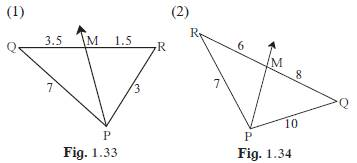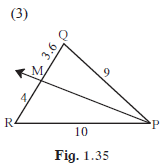Theorem: The bisector of an angle of a triangle divides the side opposite to the angle in the ratio of the remaining sides.

Therefore, we’ll find the ratio for all the triangle.Hence, for

(1)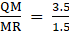= 2.33

And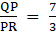= 2.33

⇒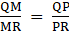⇒ In (1), ray PM is a bisector.

(2)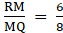= 0.75

And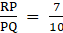= 0.7

⇒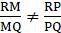⇒ In (2), ray PM is not a bisector.

(3)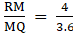= 1.1

And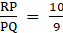= 1.11

⇒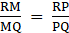⇒ In (3), ray PM is a bisector.

Question 2.

In Δ PQR, PM = 15, PQ = 25, PR = 20, NR = 8. State whether line NM is parallel to side RQ. Give reason.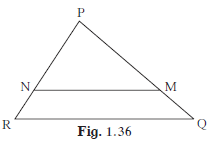By Converse of basic Proportionality Theorem

(Theorem: If a line divides any two sides of a triangle in the same ratio, then the line is parallel to the third side.)

⇒ If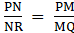, then line NM is parallel to side RQ.

∴ we’ll check if.

⇒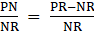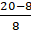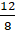And,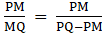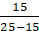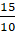⇒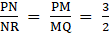, therefore line NM || side RQ

Question 3.

In Δ MNP, NQ is a bisector of ∠N. If MN = 5, PN = 7 MQ = 2.5 then find QP.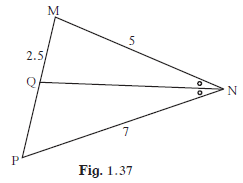Theorem: The bisector of an angle of a triangle divides the side opposite to the angle in the ratio of the remaining sides.

⇒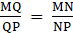⇒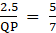⇒ QP× 5 = 2.5× 7

⇒ QP =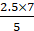⇒ QP = 3.5

Question 4.

Measures of some angles in the figure are given. Prove that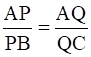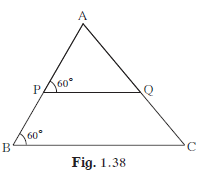Here, PQ||BC (∵ ∠ APQ≅ ∠ ABC)

(PROPERTY: If a transversal intersects two lines so that corresponding angles are congruent, then the lines are parallel)

∴ By Basic Proportionality Theorem

(Theorem : If a line parallel to a side of a triangle intersects the remaining sides in two distinct points, then the line divides the sides in the same proportion.)

⇒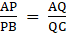Question 5.

In trapezium ABCD, side AB || side PQ || side DC, AP = 15, PD = 12, QC = 14, find BQ.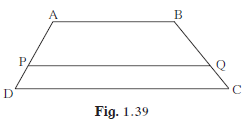By Basic Proportionality Theorem

(Theorem : If a line parallel to a side of a triangle intersects the remaining sides in two distinct points, then the line divides the sides in the same proportion.)

⇒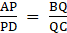⇒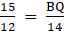⇒ BQ =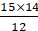⇒ BQ = 17.5

Question 6.

Find QP using given information in the figure.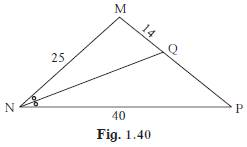Theorem: The bisector of an angle of a triangle divides the side opposite to the angle in the ratio of the remaining sides.

And ∵ NQ is angle bisector of ∠N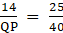⇒ QP =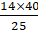⇒ QP = 22.4

Question 7.

In figure 1.41, if AB || CD || FE then find x and AE.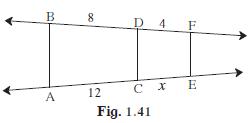Theorem: The ratio of the intercepts made on a transversal by three parallel lines is equal to the ratio of the corresponding intercepts made on any other transversal by the same parallel lines.

⇒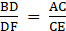⇒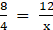⇒ x =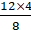⇒ x = 6

Now,AE = AC + CE

= 12 + x

= 12 + 6

⇒ AE = 18

Question 8.

In Δ LMN, ray MT bisects ∠ LMN If LM = 6, MN = 10, TN = 8, then find LT.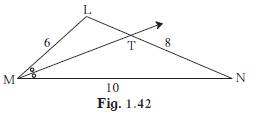Theorem: The bisector of an angle of a triangle divides the side opposite to the angle in the ratio of the remaining sides.

⇒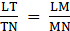⇒ LT =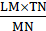⇒ LT =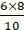⇒ LT = 4.8

Question 9.

In Δ ABC, seg BD bisects ∠ ABC. If AB = x, BC = x + 5, AD = x – 2, DC = x + 2, then find the value of x.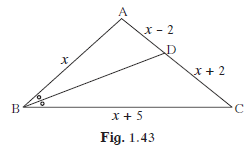Theorem: The bisector of an angle of a triangle divides the side opposite to the angle in the ratio of the remaining sides.

⇒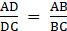⇒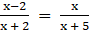⇒ x(x + 2) = (x-2)(x + 5)

⇒ x2 + 2x = x2-2x + 5x-10

⇒ x2 + 2x-x2 + 2x-5x + 10 = 0

⇒ x = 10

Question 10.

In the figure 1.44, X is any point in the interior of triangle. Point X is joined to vertices of triangle. Seg PQ || seg DE, seg QR || seg EF. Fill in the blanks to prove that, seg PR || seg DF.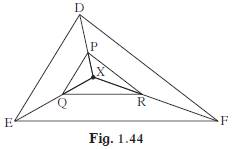Proof : IN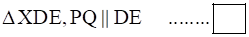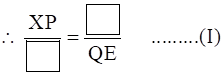(Basic proportionality theorem)

In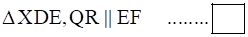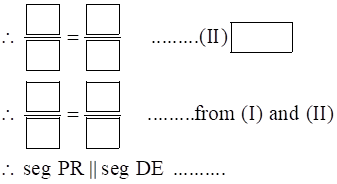(converse of basic proportionality theorem)

Proof: In ΔXDE, PQ||DE….. (Given)

∴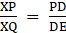…..(I)

(Basic proportionality theorem)

In ΔXDE, QR||EF …….(Given)

∴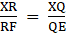………(II) (Basic Proportionality Theorem)

∴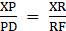……… from (I) and (II)

∴ seg PR||Seg DE ………..

(converse of basic proportionality theorem)

Question 11.

In ΔABC, ray BD bisects ∠ABC and ray CE bisects ∠ACB. If seg AB ≅ seg AC then prove that ED || BC.

PROOF: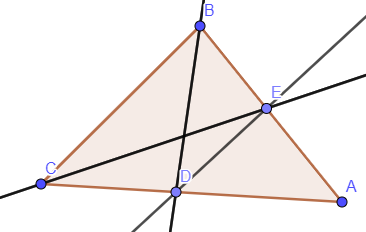Theorem: The bisector of an angle of a triangle divides the side opposite to the angle in the ratio of the remaining sides.

⇒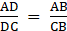……(1)

and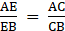…..(2) (∵ , BD and CE are angle bisectors of ∠B and ∠C respectively.)

Now, ∵ seg AB ≅ seg AC

⇒ AB = AC

⇒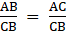⇒ R.H.S of (1) & (2) are equal.

⇒ L.H.S of (1) & (2) will be equal.

∴ Equating L.H.S of (1) &(2), we get-

⇒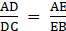⇒ ED||BC (By converse basic proportionality theorem)